# How to calculate the cubic capacity of a timber: an action algorithm

Wood is still a popular building material. For the purchase of timber or wood moldings for the construction of a profitable, it is necessary to make the correct calculation. The difficulty lies in the fact that in the sawmill and in the specialized shop the sellers use such a unit of measurement as a square meter.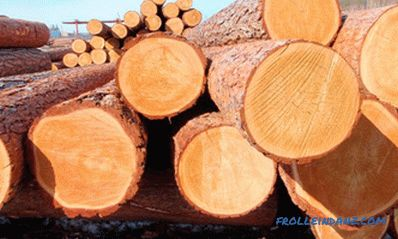Different batches of the timber may differ in the nuances of processing, so products made from them will have differences that affect the final result.

It is difficult for a non-specialist to understand how to calculate the cubic capacity correctly so that then during the work there is no need to purchase the missing material or, after construction, get rid of the excess, lamenting the money spent.

## Construction project and calculation of the cubic capacity of sawn timber

The exact cubic capacity of a timber can be accurately calculated only taking into account the features of the future building . In particular, the algorithm of actions may be as follows (features of window and door openings are not taken into account):

1. Calculate the perimeter of the walls of the structure, add length and width indicators and multiply the resulting amount by 2. If there are additional elements the structures for which the timber is used are additionally summed up and its dimensions.
2. Multiply the perimeter index by the ceiling height indicator (floor).
3. Approximately half of the material used for the construction of two walls will go to the gable.
4. The result is multiplied by the measure of the thickness of the timber used. The data obtained correspond to the volume of timber that should be purchased. These data are supplemented by another 20% margin.
5. To calculate the quantity of timber in pieces, the total amount of material needed is divided by the indicator of its volume as lumber.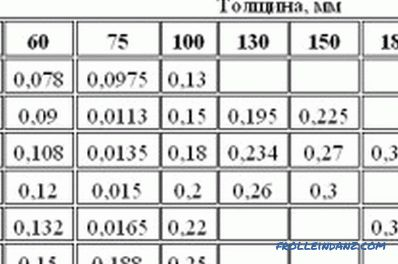Table for calculating the cubic capacity of a log and a bar.

As an example of the implementation of the proposed algorithm, we consider the calculation of the cubic capacity of a beam for the construction of a one-story house with dimensions of 5x6 m and a partition of 5 m. The ceiling height is 3 m.

Experts recommend house, which is operated only in the summer, choose a bar having a cross section of 100x100 (in millimeters). The building for permanent residence is built from a material having a large width - approximately 150 mm. In the latter case, the calculations must be supplemented by the cost of insulation, the thickness of which should be from 100 to 150 mm.

Thus, we get the following result:

• perimeter: (5 + 6) x2 + 5 = 27 m;
• the volume of all the walls of the floor: 27x3x0.1 = 8.1 or 27x3x0.15 = 12.15 (in cubic meters);
• pediment: 5x3x0.1 = 1.5 or 5x3x0.15 = 2.25 (in cubic meters);
• total: 8.1 + 1.5 + 1.92 = 11.52 or 12.15 + 2.25 + 2.88 = 17.28.

## Determining the volume of a small amount of workpiece

For the construction of the building, sawn timber having different volumes (we are talking about length, section width, height) is used, which must also be taken into account. It is important to pay attention to the unit of measurement specified in the price list. Most often, measurements are made in millimeters, but other options are possible.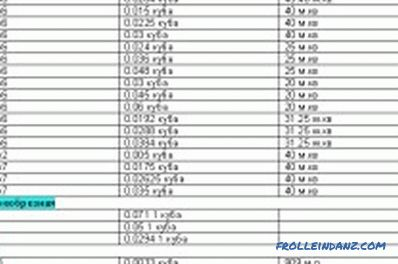Table for calculating the cubic capacity of a log and bar.

If the parameters 150x150 are indicated on the price list of the timber, the workpiece has a square cross section, the side of which is equal to 150 mm. It is quite easy to calculate the cubic capacity of such a bar, taking into account the length of 6 m. To begin with, millimeters are converted into meters (150 mm is 0.15 m), then the width is multiplied by the height and length of the beam, obtaining the following data: 0.15 x 0.15 x 5 = 0.135 m³.

If it is necessary to calculate the volumes of all the material for construction, the resulting indicator is multiplied by the number of blanks in pieces. For example, the cubic capacity of 20 pieces of timber with a section of 150x150 will be 2.7 m³.

Accordingly, the calculation of the quantity of purchased timber is made as follows: the volume indicator calculated by the method described above is divided by the volume of one product.

You can do even simpler, using ready-made calculations. With a bar length of 6 m, its amount in a cube (pieces) and the volume of a single product (cubic meters) can be: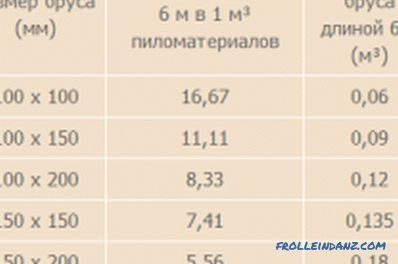Calculation table of a clean cut timber.

• 100x100 - 16.6 - 0.06;
• 100x150 - 11.1 - 0.09;
• 100x200 - 8.3 - 0.12;
• 150x150 - 7.4 - 0.135;
• 150x200 - 5.5 - 0.18;
• 200x200 - 4.1 - 0.24.

For a bar whose length is 7 meters, the indicators are as follows:

• 100x100 - 14.28 - 0.07;
• 100x150 - 9, 52 - 0.105;
• 100x200 - 7.14 - 0.14;
• 150x150 - 6.35 - 0.1575;
• 150x200 - 4.76 - 0, 21;
• 200x200 - 4.76 - 0.28.

## Calculations of lumber for large batches

There is another way to calculate the cubic capacity acceptable for large volumes of materials having different lengths and dimensions of the section.

To do this, you need to understand the difference between the "cubic meter of solid wood" and the "folding cubic meter."

The first concept is used to denote the volume of pure wood obtained by measuring individual products, excluding voids.

The cube meter is called the overall size of the stack, including voids. To determine it, the timber is pre-folded into a pile, the length of which does not exceed the length of the base material. You can dock some short items. Then measure the overall height, width and length, and the resulting figures are multiplied.

In the next stage, to obtain a cubic capacity of solid wood, the index of the storage cubic capacity is multiplied by a special conversion factor, which is regulated by state standards, for example, 6564-84, 6782. 1-75, 6782. 2-75, 13-24-86.

When calculating it is necessary to take into account that very rarely 1 cube will contain an equal number of products, and there may be several defective pieces in a batch.

Different batches may differ in the nuances of processing, so the products from them will have differences that affect the final result. So, significant difficulties may arise in the process of docking.

•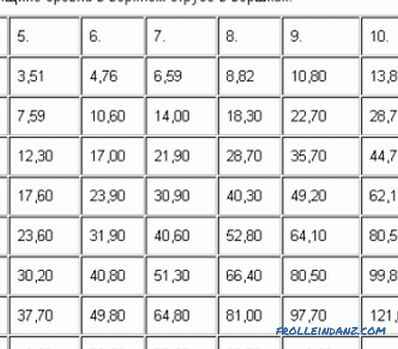Phased calculation of lumber

Calculation of lumber and its subtleties. What are the most commonly used lumber and how to distinguish them? The phased description of the compilation of the calculation table. Additional nuances of calculation.

•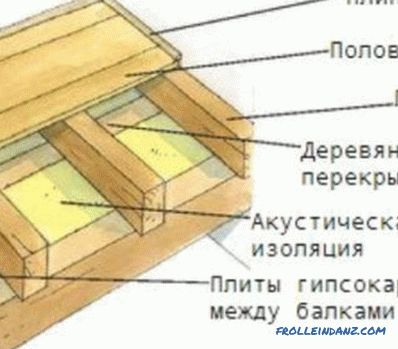The optimal lag for the floor is

Information on how to determine the step lag for the floor when using different types and sizes of floor coverings. Features of the choice of material for the lag. Installation lag on various bases.